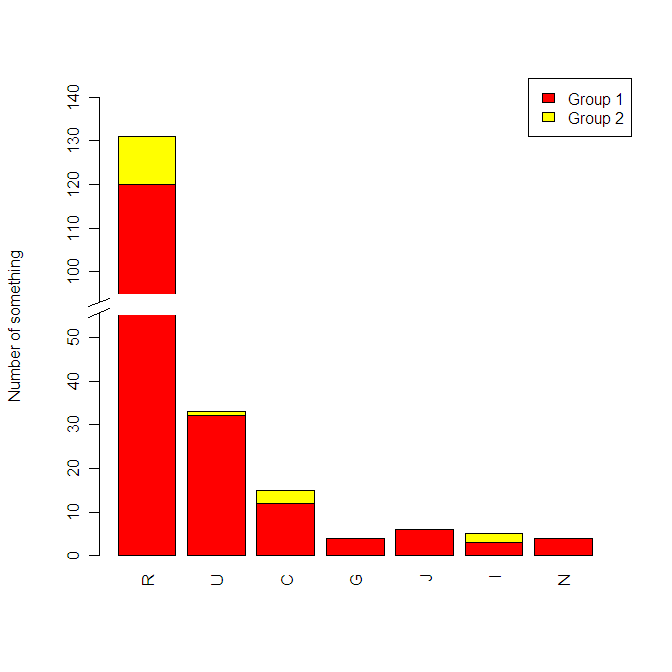# How to make a bar graph with a split Y axis in R

There is a function, gap.barplot for doing that in the plotrix package, but I was not happy with the way the result looked, so I started out making my own way using subplot in the TeachingDemos library. I have gutted the two functions I actually used from TeachingDemos to just get the functionality I need for this plot:

```
# dataset:
data=data.frame(R=c(120,11),U=c(32,1),C=c(12,3),G=c(4,0),J=c(6,0),I=c(3,2),N=c(4,0))
# I want to plot the lower values up to 55, then a split to 95 for the
# last top. This should make it clear which is the highest, without
# drowning out the other data.

# I want the split to be approx 5% of the scale,

# as I am to plot the ranges 0 - 55 and 95 - 140, in total 10 decades,
lower=c(0,55)
upper=c(95,140)
# This is 10 decades. I multiply that with 2 and add 5% and get 21 units on the outer
# Y axis:
y_outer=21

lowspan=c(0,11)
topspan=c(lowspan+1,21)

ylabel="Number of something"
legendtext=c('Group 1','Group 2')

cnvrt.coords <-function(x,y=NULL){
# Stolen from the teachingDemos library, simplified for this use case
xy <- xy.coords(x,y, recycle=TRUE)
cusr <- par('usr')
cplt <- par('plt')
plt <- list()
plt\$x <- (xy\$x-cusr)/(cusr-cusr)
plt\$y <- (xy\$y-cusr)/(cusr-cusr)
fig <- list()
fig\$x <- plt\$x*(cplt-cplt)+cplt
fig\$y <- plt\$y*(cplt-cplt)+cplt
return( list(fig=fig) )
}

subplot <- function(fun, x, y=NULL){
# Stolen from the teachingDemos library, simplified for this use case
on.exit(par(old.par))
xy <- xy.coords(x,y)
xy <- cnvrt.coords(xy)\$fig
par(plt=c(xy\$x,xy\$y), new=TRUE)
fun
return(invisible(tmp.par))
}

##############################################
#
#
# The main program starts here:
#
#

# Setting up an outer wireframe for the plots.
plot(c(0,1),c(0,y_outer),type='n',axes=FALSE,ylab=ylabel,xlab='')
# Plotting the lower range in the lower 11/21 of the plot.
# xpd=FALSE to clip the bars
subplot(barplot(as.matrix(data),col=heat.colors(2),ylim=lower,xpd=FALSE,las=3),x=c(0,1),y=lowspan)

# Plotting the upper range in the upper 9/21 of the plot, 1/21 left to
# the split. Again xpd=FALSE, names.arg is set up to avoid having
# the names plotted here, must be some easier way to do this but
# this works
subplot(barplot(as.matrix(data),col=heat.colors(2),ylim=upper,xpd=FALSE,names.arg=vector(mode="character",length=length(data))), x=c(0,1),y=topspan)

# Legend. An annoiance is that the colors comes in the opposite
# order than in the plot.
legend("topright",legendtext,fill=heat.colors(2))

# so far so good. (Just run the upper part to see the result so far)
# Just want to make the ends of the axes a bit nicer.
# All the following plots are in units of the outer coordinate system

lowertop=lowspan+0.1     # Where to end the lower axis
breakheight=0.5   # Height of the break
upperbot=lowertop+breakheight # Where to start the upper axes
markerheight=0.4 # Heightdifference for the break markers
markerwidth=.04  # With of the break markers

# Draw the break markers:
lines(c(0,0),c(1,lowertop))
lines(c(markerwidth/-2,markerwidth/2),c(lowertop-markerheight/2,lowertop+markerheight/2))
lines(c(0,0),c(upperbot,14))
lines(c(markerwidth/-2,markerwidth/2),c(upperbot-markerheight/2,upperbot+markerheight/2))

```